##### Actions

(diff) ← Older revision | Latest revision (diff) | Newer revision → (diff)

An element of an extension of the field of rational numbers (cf. Extension of a field) based on the divisibility of integers by a given prime number. The extension is obtained by completing the field of rational numbers with respect to a non-Archimedean valuation (cf. Norm on a field).

A-adic integer, for an arbitrary prime number, is a sequence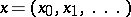of residues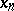modulo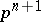which satisfy the condition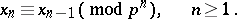The addition and the multiplication of-adic integers is defined by the formulas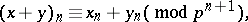Each integeris identified with the-adic number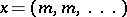. With respect to addition and multiplication, the-adic integers form a ring which contains the ring of integers. The ring of-adic integers may also be defined as the projective limitof residues modulo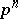(with respect to the natural projections).

A-adic number, or rational-adic number, is an element of the quotient field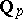of the ring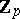of-adic integers. This field is called the field of-adic numbers and it contains the field of rational numbers as a subfield. Both the ring and the field of-adic numbers carry a natural topology. This topology may be defined by a metric connected with the-adic norm, i.e. with the function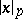of the-adic numberwhich is defined as follows. If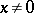,can be uniquely represented as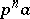, whereis an invertible element of the ring of-adic integers. The-adic normis then equal to. If, then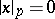. Ifis initially defined on rational numbers only, the field of-adic numbers can be obtained as the completion of the field of rational numbers with respect to the-adic norm.

Each element of the field of-adic numbers may be represented in the form(*)

whereare integers,is some integer,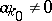, and the series (*) converges in the metric of the field. The numbers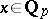with(i.e.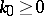) form the ringof-adic integers, which is the completion of the ring of integers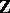of the field. The numbers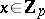with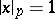(i.e.,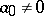) form a multiplicative group and are called-adic units. The set of numberswith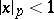(i.e.) forms a principal ideal inwith generating element. The ringis a complete discrete valuation ring (cf. also Discretely-normed ring). The fieldis locally compact in the topology induced by the metric. It therefore admits an invariant measure, usually taken with the condition. For different, the valuationsare independent, and the fieldsare non-isomorphic. Numerous facts and concepts of classical analysis can be generalized to the case of-adic fields.-adic numbers are connected with the solution of Diophantine equations modulo increasing powers of a prime number. Thus, if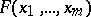is a polynomial with integral coefficients, the solvability, for all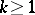, of the congruence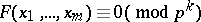is equivalent to the solvability of the equation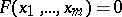in-adic integers. A necessary condition for the solvability of this equation in integers or in rational numbers is its solvability in the rings or, correspondingly, in the fields of-adic numbers for all. Such an approach to the solution of Diophantine equations and, in particular, the question whether these conditions — the so-called local conditions — are sufficient, constitutes an important branch of modern number theory (cf. Diophantine geometry).

The above solvability condition may in one special case be replaced by a simpler one. In fact, if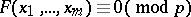has a solution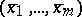and if this solution defines a non-singular point of the hypersurface, whereis the polynomialmodulo, then this equation has a solution in-adic integers which is congruent tomodulo. This theorem, which is known as the Hensel lemma, is a special case of a more general fact in the theory of schemes.

The ring of-adic integers may be regarded as a special case of the construction of Witt rings. The ring of-adic integers is obtained ifis the finite field ofelements (cf. Witt vector). Another generalization of-adic numbers are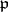-adic numbers, resulting from the completion of algebraic number fields with respect to non-Archimedean valuations connected with prime divisors.-adic numbers were introduced by K. Hensel . Their canonical representation (*) is analogous to the expansion of analytic functions into power series. This is one of the manifestations of the analogy between algebraic numbers and algebraic functions.

How to Cite This Entry: